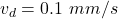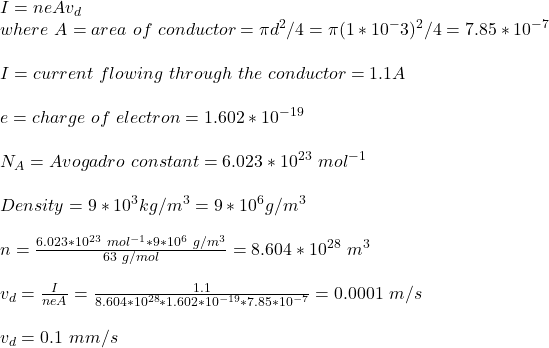## 3. Assume that each atom of copper contributes one free electron. The density of Cu is 9g/cc and atomic weight is 63g. if the current flowin

Question

3. Assume that each atom of copper contributes one free electron. The density of Cu is 9g/cc and atomic weight is 63g. if the current flowing through the copper wire of 1mm dia is 1.1A, drift velocity of electrons will be-

in progress 0
5 months 2021-08-14T00:46:07+00:00 1 Answers 12 views 0